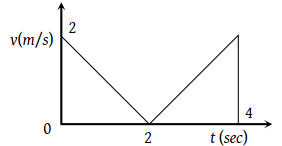A bird flies for 4 s with a velocity of $|\text{\hspace{0.17em}}t-2|\text{\hspace{0.17em}}m/s$ in a straight line, where t is time in seconds. It covers a distance of

(1) 2 m

(2) 4 m

(3) 6 m

(4) 8 m

Concept Videos :-

#4-Solved Examples 1
#5-Solved-Examples-2
#6-Solved-Examples-3
#7-Solved-Examples-4
#1-Understanding Position
#2-Understanding Path Length
#3-Understanding Displacement
#20-Graph x vs t
#21- v vs t and a vs t graph
#22-Solved-Examples-12
#23-Solved-Examples-13
#24-Solved-Examples-14
#25-Solved-Examples-15

Concept Questions :-

Distance and displacement
Graphs

(2) The velocity time graph for given problem is shown in the figure.Distance travelled S = Area under curve= 2 + 2 = 4m

Difficulty Level:

• 13%
• 47%
• 15%
• 27%